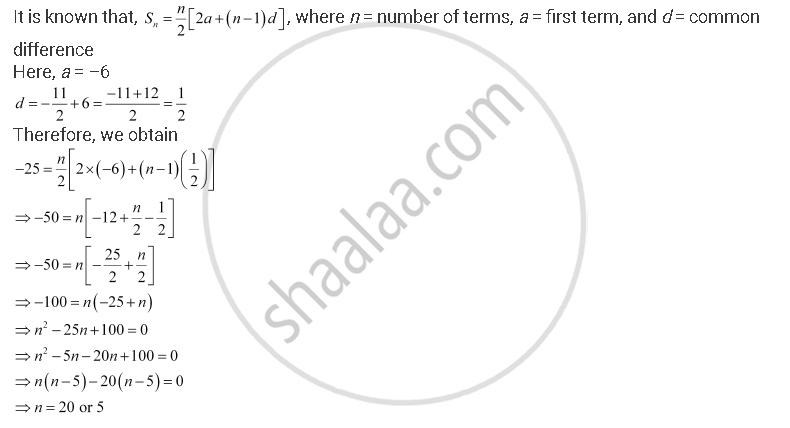CBSE (Arts) Class 11CBSE
Share

# How Many Terms of the A.P. -6 , -11/2 , -5... Are Needed to Give the Sum –25? - CBSE (Arts) Class 11 - Mathematics

ConceptArithmetic Progression (A.P.)

#### Question

How many terms of the A.P.  -6 , -11/2 , -5... are needed to give the sum –25?

#### Solution

Let the sum of n terms of the given A.P. be –25.Is there an error in this question or solution?

#### APPEARS IN

NCERT Solution for Mathematics Textbook for Class 11 (2018 to Current)
Chapter 9: Sequences and Series
Q: 4 | Page no. 185

#### Video TutorialsVIEW ALL 

Solution How Many Terms of the A.P. -6 , -11/2 , -5... Are Needed to Give the Sum –25? Concept: Arithmetic Progression (A.P.).
S